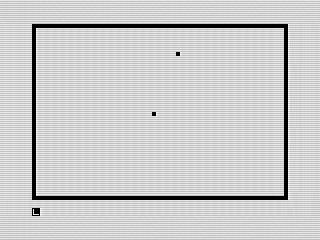February’s Program: Golf

Play 18 holes of golf in this simple graphic/physics game.This month’s program is a simple game of Golf. Although not overly exciting, it does show off a few ZX81 BASIC features.

One oddity of the ZX81 is that π (pi) is built in, something I haven’t seen in other languages. In this case, it is used to calculate the direction of the golf ball. I also used the PLOT and UNPLOT commands in this program, something I generally avoided due to speed constraints.

If you take a look at the listing, you’ll notice lines like this:

130 LET A1=(A1<1)+(62 AND A1>62)+(A1 AND (A1>=1 AND A1<=62))
140 LET B1=(B1<1)+(42 AND B1>2)+(B1 AND (B1>=1 AND B1<=42))

Although ZX81 BASIC didn’t have an ELSE or SWITCH or the ability to execute multiple lines of code within an IF statement, it did however understand boolean logic. You would get 1 if true, and 0 if false. The lines above are using this to validate if the ball is out of bounds and, if so, ensure it stays on the screen. The A1<1 for instance would return 1 if the ball was less than 1, keeping it on the border. Otherwise, it is 0. This is added to the next check, (62 AND A1>62). Now, in this case, if a constant or variable is passed, it returns that number. That is if A1>62, then it returns 62. Neat, huh? The last part returns the value of A1 if it is inside the bounds. This use of boolean can be used in anywhere a number can be. Although simple in many ways, ZX81 BASIC offered some unique features that more advanced languages lack. Until next month, keep programing!